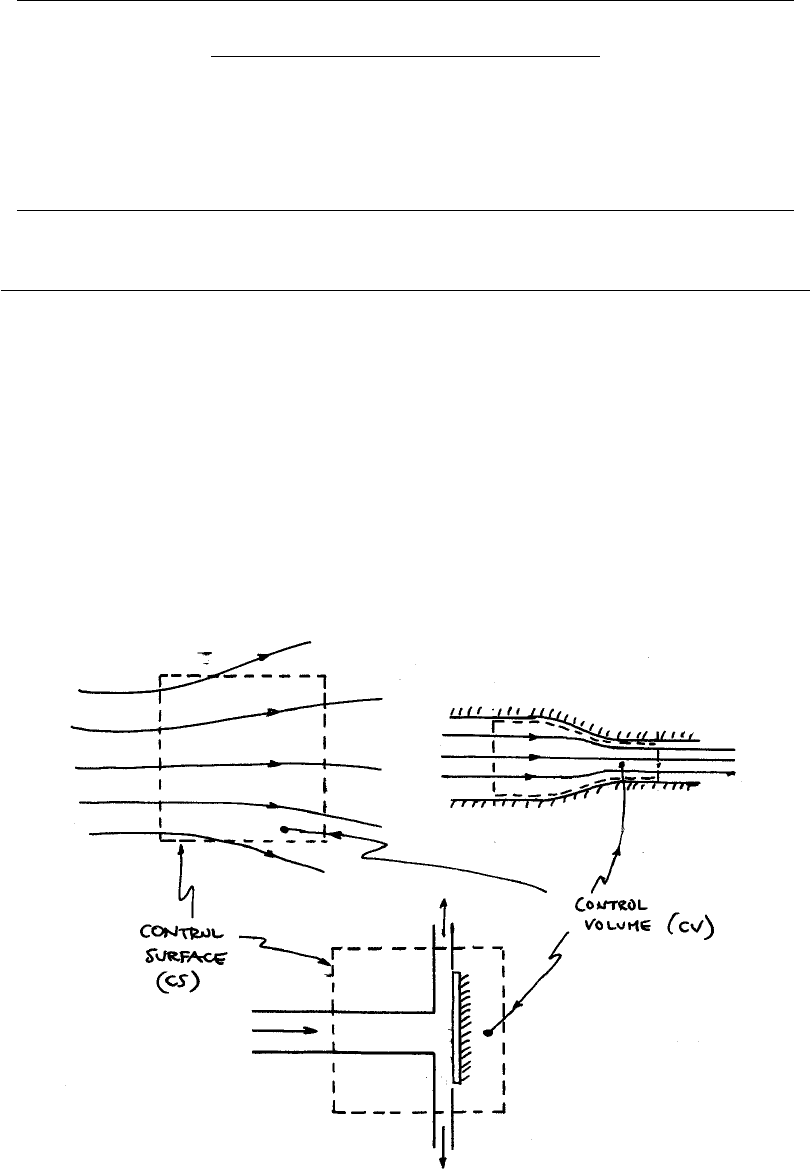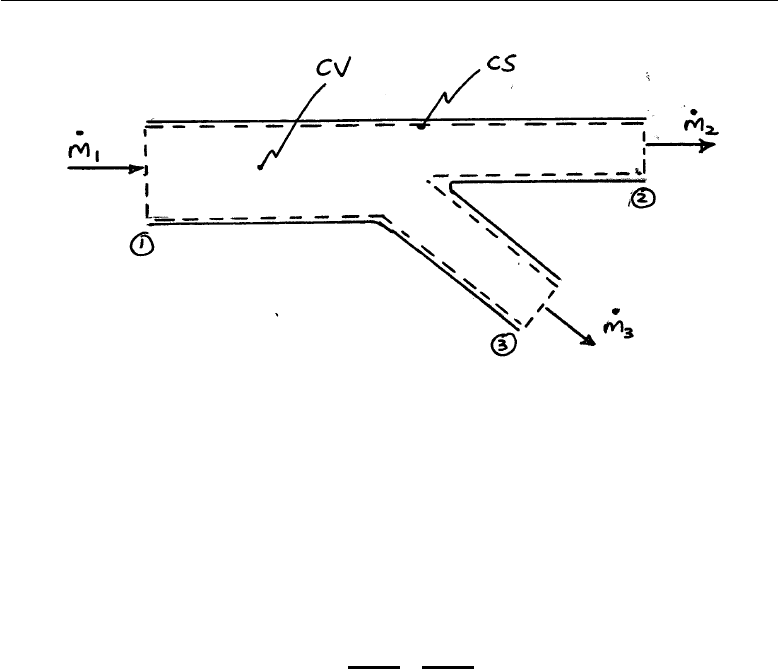# ENGR2000 Lecture Notes - Lecture 3: Trac, Reynolds Transport Theorem, Curtin University

22 views17 pages
School
Department
Course
Professor2nd-Year Fluid Mechanics, Faculty of Science & Engineering, Curtin University
ENGR2000: FLUID MECHANICS
For Second-year Chemical, Petroleum, Civil & Mechanical Engineering
FLUID MECHANICS LECTURE NOTES
CHAPTER 3: MASS CONSERVATION, MOMENTUM AND ENERGY
3.1 Introduction
In order to introduce the ideas of conservation, it is often necessary to isolate
a part of the system and consider what is going on within that part of the
ﬂow ﬁeld. This is achieved by using a control volume (CV). We enclose a
part of the whole system by a notional boundary and perform our analysis
using the CV – what is happening within it and what is happening at its
boundaries. Examples of CV’s are seen in Fig. 3.1.
FIGURE 3.1: Examples of control volumes
Chapter 3 Page 1
find more resources at oneclass.com
find more resources at oneclass.com
Unlock document

This preview shows pages 1-3 of the document.
Unlock all 17 pages and 3 million more documents.2nd-Year Fluid Mechanics, Faculty of Science & Engineering, Curtin University
Note that a CV can include regions where there is no ﬂuid. The CV surface
- called the Control Surface (CS) - should not cross solid boundaries nor,
strictly, include solid components; however, we can ‘isolate’ such elements by
putting a separate CS around them. Often (see lower example in Fig. 3.1)
we do not bother to draw this isolating boundary in since it does not aﬀect
the analysis.
The CV’s illustrated above are stationary; we can use moving CV’s – would
these be of greater use? In mathematical terminology should we use an
Eulerian (ﬁxed frame) or Lagrangian (moving frame) approach to under-
standing ﬂow behaviour? Consider the following analogy to ﬂuid ﬂow: Traﬃc
is travelling along a road and we wish to characterise it. The Eulerian would
set up a check-point at a particular location and measure the number of cars
per hour passing that point. In contrast, the Lagrangian would follow partic-
ular vehicles and determine their destinations. Each is valid form of analysis
meeting diﬀerent interests.
In Fluid Mechanics, we are not generally interested in the fate of a particular
‘lump’ of ﬂuid and so we adopt a Eulerian approach. In Solid Mechanics the
Lagrangian approach is used because we are concerned with the motion (eg.
locii and orbits) of particular parts of the structure.
3.2 Mass conservation
The principle of mass conservation is based upon the physical recognition that
mass is neither created nor destroyed (certainly in the Newtonian world!).
In Fluid Mechanics, this is interpreted in terms of mass ﬂow. For a control
volume in which the mean ﬂow ﬁeld is time independent, we can say that
the rate of mass ﬂow into a CV must be equal to the rate at which it leaves.
To illustrate this idea, consider Fig. 3.X1.
Chapter 3 Page 2
find more resources at oneclass.com
find more resources at oneclass.com
Unlock document

This preview shows pages 1-3 of the document.
Unlock all 17 pages and 3 million more documents.2nd-Year Fluid Mechanics, Faculty of Science & Engineering, Curtin University
FIGURE 3.X1: Fluid mass ﬂow through a simple pipe system
Denoting a mass ﬂow-rate as ˙m(the over-dot means ’rate with time’, i.e. the
time derivative) with units kg/s, then it is obvious that
˙m1= ˙m2+ ˙m3(3.X1)
to show that this is a conservation principle, re-write it as
0 = ˙m1˙m2˙m3
|{z }
Net mass ﬂow-rate into CV
(3.X2)
Thus the net mass ﬂow rate into (or out of) the CV is zero so no ﬂuid mass
is ‘being created’ inside the CV.
With the above ideas in mind, we now undertake a general analysis of mass
conservation that can be applied to any ﬂow ﬁeld.
For simplicity we shall consider a two-dimensional ﬂow. We set up a CV in
the ﬂow as shown in Fig. 3.2. To make life easy we choose a square CV with
sides in the x- and y-directions. The choice of CV can be very important in
facilitating the analysis.
Chapter 3 Page 3
find more resources at oneclass.com
find more resources at oneclass.com
Unlock document

This preview shows pages 1-3 of the document.
Unlock all 17 pages and 3 million more documents.